> 文档中心 > 『递归』递归概念与典型实例

# 『递归』递归概念与典型实例

👨‍🎓作者简介：一位喜欢写作，计科专业大二菜鸟

🏡个人主页：starry陆离

🕒首发日期：2022年8月2日星期二

🌌上期文章：『算法导论』什么是算法？什么是程序？

📚订阅专栏：『算法分析与设计』
🍁每日推荐：『牛客网-面试神器』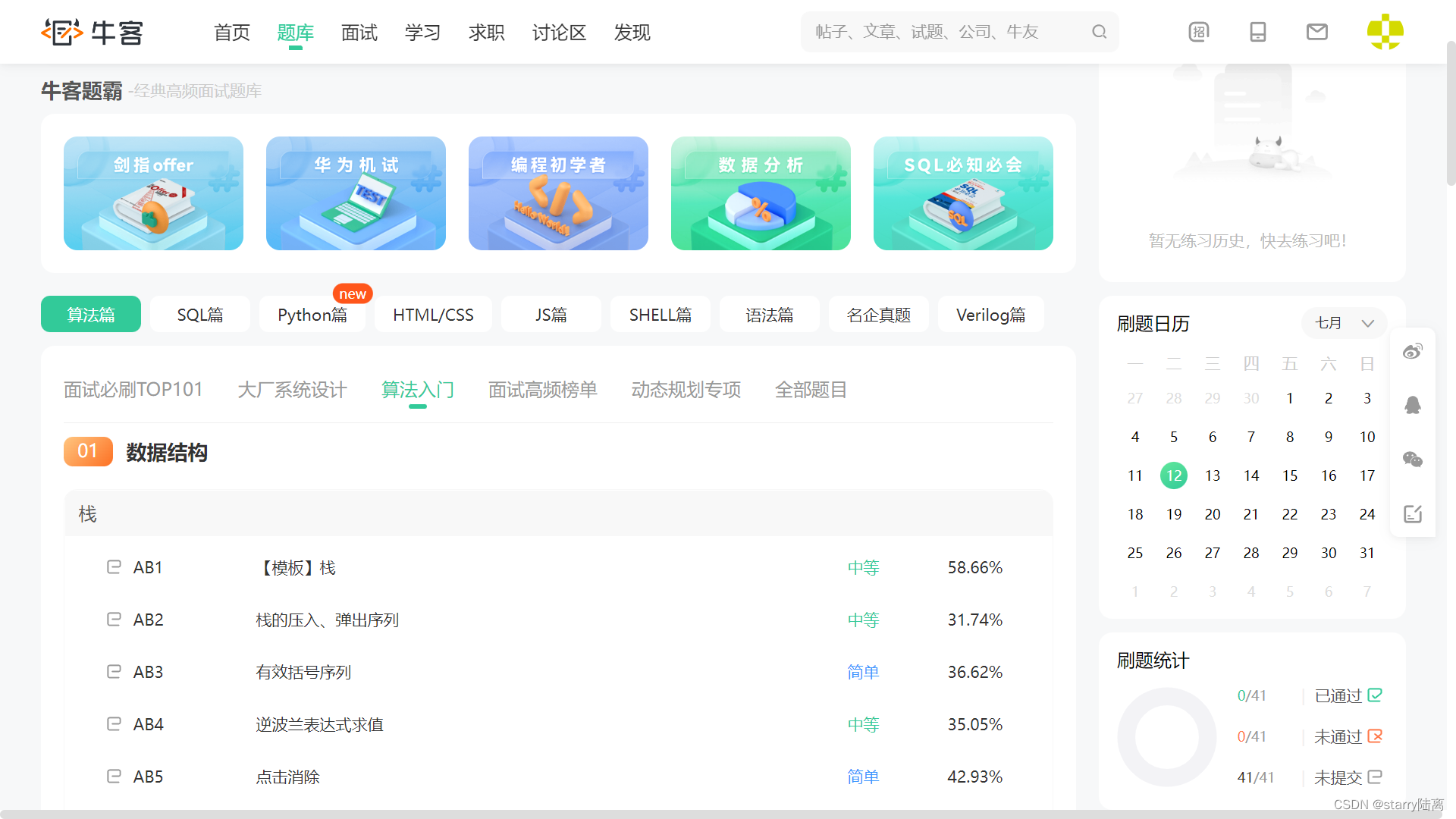### 这里写目录标题

• 1.引言
• 2.递归的定义
• 3.递归的要素
• 4.递归特点
• 5.递归的特点
• 6.递归的优缺点
• 7.典型递归实例
• 7.1求阶乘
• 7.2Fibonacci数列
• 7.3青蛙跳台阶

@[TOC](『递归概念与典型实例』)

# 1.引言

``伪代码：for i=1 to 100   sum = sum + i``

``sum(n)表示1…n的和 sum(100) = sum(99) + 100  = sum(98) + 99 + 100  = ……  = sum(1) + 2 + 3 + 4 + …… + 99 + 100  = 1 + 2 + 3 + 4 +…… + 99 + 100``

# 2.递归的定义

• 若p函数定义中调用p函数，称之为直接递归

• 若p函数定义中调用q函数，而q函数定义中又调用p函数，称之为间接递归

# 3.递归的要素

1、递归表达式 （递归方程）

2、递归结束条件 （边界条件）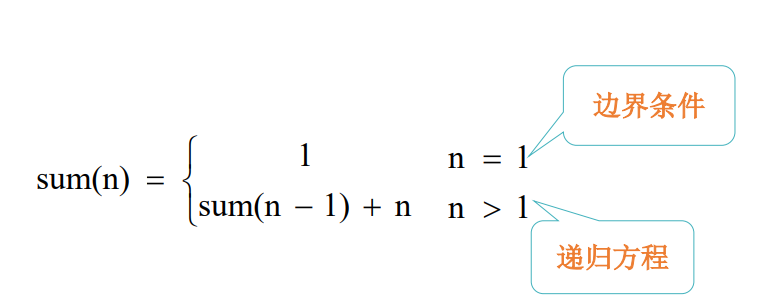``int sum(int n){if(n==1)return 1;//递归结束条件else sum(n-1)+n;//递归表达式}``

# 4.递归特点

(1) 需要解决的问题可以转化为一个或多个子问题来求解，而这些子问题的 求解方法与原问题完全相同，只是在数量规模上不同

(2) 递归调用的次数必须是有限的

(3) 必须有结束递归的条件来终止递归

# 5.递归的特点

(1) 定义是递归的（阶乘、斐波那契数列等）

(2) 数据结构是递归的（单链表、二叉树等）

(3) 问题的求解方法是递归的（汉诺塔、回溯法等）

# 7.典型递归实例

## 7.1求阶乘

n!=1×2×3×……×n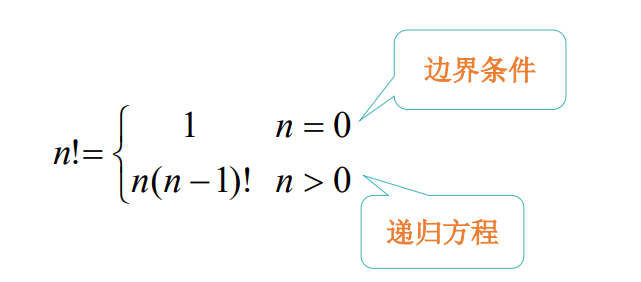``int f(int n){if(n==1)return 1;//递归结束条件else n*f(n-1);//递归表达式}``

## 7.2Fibonacci数列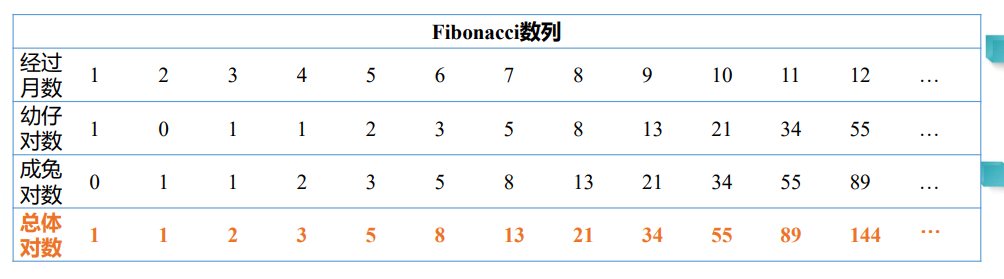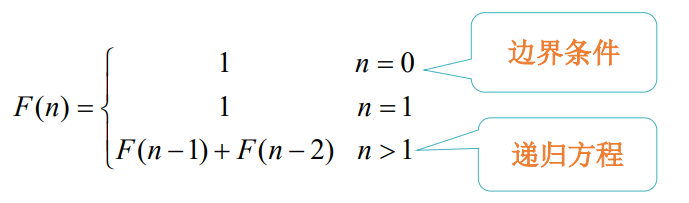``int fibonacci(int n){if(n<=1)return 1;//递归结束条件    return fibonacci(n-1)+fibonacci(n-2);//递归表达式}``

## 7.3青蛙跳台阶

• 求该青蛙跳上一个n级的台阶总共有多少种跳法？

🍁每日推荐：基础算法无论在研究生面试还是求职面试都是十分重要的一环，这里推荐一款算法面试神器：牛客网-面试神器；算法题只有多刷勤刷才能保持思路与手感，大家赶紧行动起来吧（温馨提示：常见的面试问答题库也很nice哦）